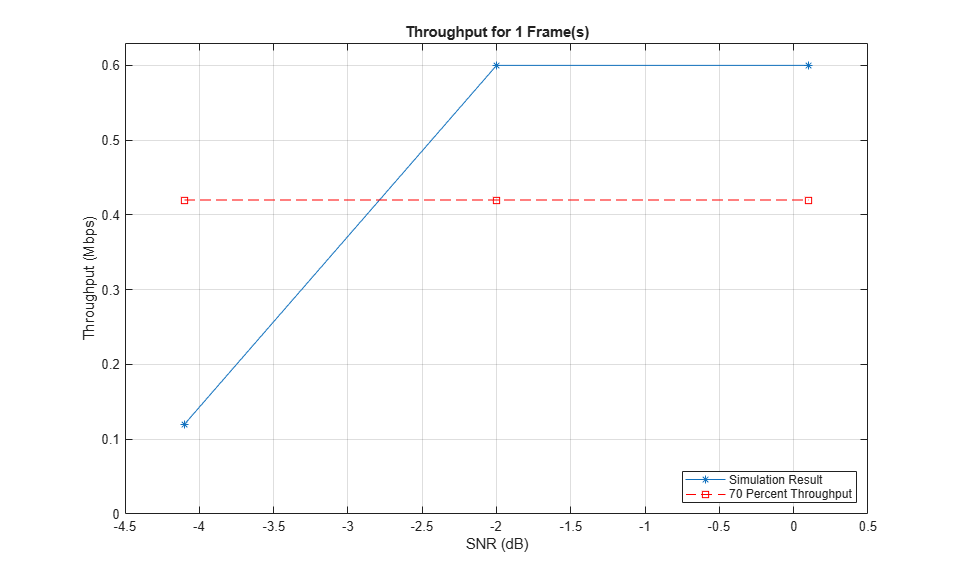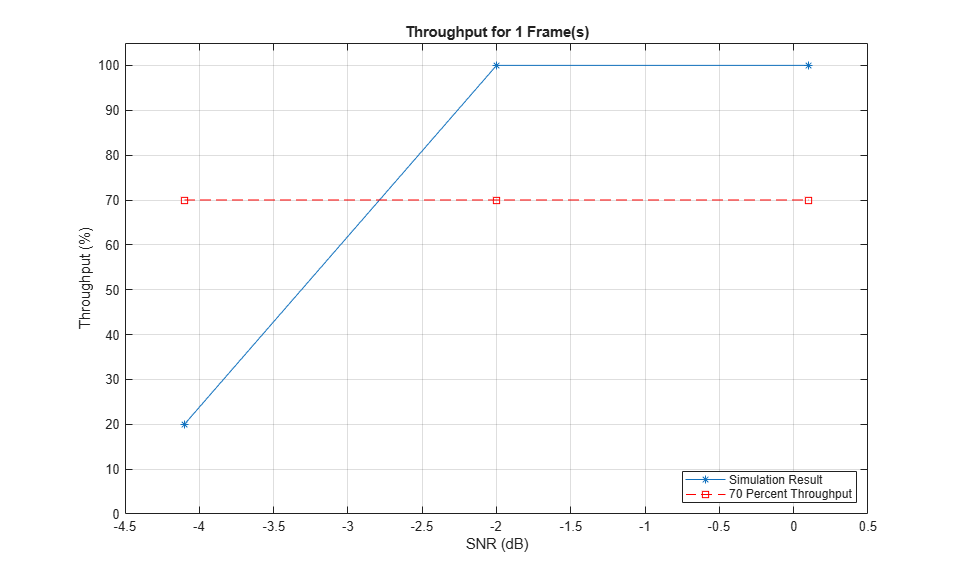Documentation

## PUSCH Throughput Conformance Test

This example demonstrates how to measure the Physical Uplink Shared Channel (PUSCH) throughput performance using the LTE Toolbox™ under conformance test conditions as defined in TS36.104: two receive antennas, normal cyclic prefix, Extended Pedestrian A (EPA5) channel, FRC A3-2 [ 1 ].

### Introduction

TS 36.104 [ 1 ] defines the performance requirements for Physical Uplink Shared Channel (PUSCH) as a minimum throughput for a given SNR assuming Hybrid Automatic Repeat reQuest (HARQ) retransmissions. This example demonstrates how the conformance test can be constructed using the LTE Toolbox.

Transmission is simulated using the Extended Pedestrian A (EPA) propagation channel model using 8 HARQ processes. Channel noise is added to the received waveform which is then SC-FDMA demodulated, resulting in a received resource grid for each receive antenna. Channel estimation is performed to determine the channel between each transmit/receive antenna pair. Minimum Mean Square Error (MMSE) equalization is performed on the received resource grid using the estimated channel to recover the resource grid. PUSCH data is then extracted and decoded from this recovered resource grid. Using the result of the block CRC, the throughput performance of the transmit/receive chain is determined.

### Simulation Configuration

The example is executed for a simulation length of 1 frame at an SNR of -4.1 dB, -2.0 dB and 0.1 dB as per TS 36.104, Table 8.2.1.1-1 [ 1 ]. A large number of `NFrames` should be used to produce meaningful throughput results. `SNRIn` can be an array of values or a scalar.

```NFrames = 1; % Number of frames to simulate at each SNR SNRIn = [-4.1, -2.0, 0.1]; % SNR points to simulate ```

### UE Configuration

User Equipment (UE) settings are specified in a structure form.

```ue.TotSubframes = 1; % Total number of subframes to generate a waveform for ue.NCellID = 10; % Cell identity ue.RC = 'A3-2'; % FRC number ```

### Propagation Channel Model Configuration

Propagation channel model characteristics are set using a structure containing the fields specified below. These are set according to TS 36.104, Table 8.2.1.1-1 [ 1 ].

```chcfg.NRxAnts = 2; % Number of receive antenna chcfg.DelayProfile = 'EPA'; % Delay profile chcfg.DopplerFreq = 5.0; % Doppler frequency chcfg.MIMOCorrelation = 'Low'; % MIMO correlation chcfg.Seed = 100; % Channel seed chcfg.NTerms = 16; % Oscillators used in fading model chcfg.ModelType = 'GMEDS'; % Rayleigh fading model type chcfg.InitPhase = 'Random'; % Random initial phases chcfg.NormalizePathGains = 'On'; % Normalize delay profile power chcfg.NormalizeTxAnts = 'On'; % Normalize for transmit antennas ```

### Channel Estimator Configuration

Channel estimation settings are defined using a structure `cec`. An EPA delay profile causes the channel response to change slowly over frequency. Therefore, a large frequency averaging window of 13 Resource Elements (REs) is used. The Demodulation Reference Signal (DRS) is contained in only one symbol per slot, therefore a time averaging window of 1 RE is used. This will not include any pilots from an adjacent slot when averaging.

```cec.PilotAverage = 'UserDefined'; % Type of pilot averaging cec.FreqWindow = 13; % Frequency averaging windows in REs cec.TimeWindow = 1; % Time averaging windows in REs cec.InterpType = 'cubic'; % Interpolation type cec.Reference = 'Antennas'; % Reference for channel estimation ```

To generate the uplink Reference Model Channel (RMC) the LTE Toolbox functions lteRMCUL and lteRMCULTool are used. lteRMCUL creates a configuration structure for given UE settings; specific to a given Fixed Reference Channel (FRC). This configuration structure is constructed as per TS36.104 Annex A [ 1 ] and is used by lteRMCULTool to generate an SC-FDMA modulated waveform. The sub-structure `PUSCH` defines the parameters associated with PUSCH; containing the vector defining the transport data capacity per subframe. These lengths are used when decoding Uplink Shared Channel (UL-SCH).

```% Generate FRC configuration structure for A3-2 frc = lteRMCUL(ue); % Transport block sizes for each subframe within a frame trBlkSizes = frc.PUSCH.TrBlkSizes; codedTrBlkSizes = frc.PUSCH.CodedTrBlkSizes; % Redundancy version sequence rvSequence = frc.PUSCH.RVSeq; ```

### Set Propagation Channel Model Sampling Rate

The sampling rate for the channel model is set using the value returned from lteSCFDMAInfo.

```info = lteSCFDMAInfo(frc); chcfg.SamplingRate = info.SamplingRate; ```

### Processing Loop

The throughput test is carried out over a number of SNR points. To determine the throughput at an SNR point, the PUSCH data is analyzed on a subframe by subframe basis using the following steps:

• Update Current HARQ Process. After every 8 subframes, the given HARQ process either carries new transport data or a retransmission of previously sent transport data depending upon the Acknowledgment (ACK) or Negative Acknowledgment (NACK) based on CRC results. All this is handled by the HARQ scheduler, hHARQScheduling.m.

• Create Transmit Waveform. Using the input data generated by the HARQ scheduler and the `frc` structure, lteRMCULTool produces an SC-FDMA modulated waveform and a populated resource grid containing the physical channels and signals.

• Noisy Channel Modeling. The waveform is passed through a fading channel and Additive White Gaussian Noise (AWGN) added.

• Perform Synchronization and SC-FDMA Demodulation. The received symbols are synchronized to account for a combination of implementation delay and channel delay spread. The symbols are then SC-FDMA demodulated.

• Perform Channel and Noise Power Spectral Density Estimation. The channel and noise power spectral density are estimated to aid in equalization and decoding.

• Perform MMSE Equalization. The channel and noise estimates are used to equalize the received PUSCH symbols.

• Decode the PUSCH. The recovered PUSCH symbols for all transmit and receive antenna pairs, along with a noise estimate, are demodulated and descrambled by ltePUSCHDecode to obtain an estimate of the received codeword.

• UL-SCH Channel Decoding._ The vector of decoded soft bits is passed to lteULSCHDecode; this decodes the codeword and returns the block CRC error and this is used to determine the throughput of the system. The contents of the new soft buffer, `harqProc(harqID).decState`, is available at the output of this function to be used for the next subframe. The transport block size is obtained from a lookup table of sizes for each subframe.

```% Initialize variables used in the simulation and analysis totalBLKCRC = zeros(numel(SNRIn), NFrames*10); % Total block CRC vector bitThroughput = zeros(numel(SNRIn), NFrames*10); % Total throughput vector resultIndex = 1; % Initialize frame counter index for SNRdB = SNRIn fprintf('\nSimulating at %g dB SNR for a total %d Frame(s)', ... SNRdB, NFrames); % Calculate required AWGN channel noise SNR = 10^(SNRdB/20); N = 1/(SNR*sqrt(double(info.Nfft)))/sqrt(2.0); rng('default'); % Store results for every subframe at SNR point bitTp = zeros(1, NFrames*10); % Intermediate bit throughput vector blkCRC = zeros(1, NFrames*10); % Intermediate block CRC vector % Initialize state of all HARQ processes harqProcesses = hNewHARQProcess(frc); % Initialize HARQ process IDs to 1 as the first non-zero transport % block will always be transmitted using the first HARQ process. This % will be updated with the full sequence output by lteRMCULTool after % the first call to the function harqProcessSequence = 1; offsetused = 0; for subframeNo = 0:(NFrames*10-1) % Update subframe number frc.NSubframe = subframeNo; % Get HARQ process ID for the subframe from HARQ process sequence harqID = harqProcessSequence(mod(subframeNo, length(harqProcessSequence))+1); % If there is a transport block scheduled in the current subframe % (indicated by non-zero 'harqID'), perform transmission and % reception. Otherwise continue to the next subframe if harqID == 0 continue; end % Update current HARQ process harqProcesses(harqID) = hHARQScheduling(... harqProcesses(harqID), subframeNo, rvSequence); % Update the PUSCH transmission config with HARQ process state frc.PUSCH = harqProcesses(harqID).txConfig; data = harqProcesses(harqID).data; % Create transmit waveform and get the HARQ scheduling ID sequence % from 'frcOut' structure output which also contains the waveform % configuration and OFDM modulation parameters [txWaveform,~,frcOut] = lteRMCULTool(frc, data); % Add 25 sample padding. This is to cover the range of delays % expected from channel modeling (a combination of % implementation delay and channel delay spread) txWaveform = [txWaveform; zeros(25, 1)]; %#ok<AGROW> % Get the HARQ ID sequence from 'frcOut' for HARQ processing harqProcessSequence = frcOut.PUSCH.HARQProcessSequence; % The initialization time for channel modeling is set each subframe % to simulate a continuously varying channel chcfg.InitTime = subframeNo/1000; % Pass data through channel model rxWaveform = lteFadingChannel(chcfg, txWaveform); % Add noise at the receiver v = N*complex(randn(size(rxWaveform)), randn(size(rxWaveform))); rxWaveform = rxWaveform+v; % Calculate synchronization offset offset = lteULFrameOffset(frc, frc.PUSCH, rxWaveform); if (offset < 25) offsetused = offset; end % SC-FDMA demodulation rxSubframe = lteSCFDMADemodulate(frc, ... rxWaveform(1+offsetused:end, :)); % Channel and noise power spectral density estimation [estChannelGrid, noiseEst] = lteULChannelEstimate(frc, ... frc.PUSCH, cec, rxSubframe); % Extract REs corresponding to the PUSCH from the given subframe % across all receive antennas and channel estimates puschIndices = ltePUSCHIndices(frc, frc.PUSCH); [puschRx, puschEstCh] = lteExtractResources( ... puschIndices, rxSubframe, estChannelGrid); % MMSE equalization rxSymbols = lteEqualizeMMSE(puschRx, puschEstCh, noiseEst); % Update frc.PUSCH to carry complete information of the UL-SCH % coding configuration trBlkSize = trBlkSizes(mod(subframeNo, 10)+1); frc.PUSCH = lteULSCHInfo(frc, frc.PUSCH, trBlkSize, 'chsconcat'); % Decode the PUSCH rxEncodedBits = ltePUSCHDecode(frc, frc.PUSCH, rxSymbols); % Decode the UL-SCH channel and store the block CRC error for given % HARQ process [rxDecodedBits, harqProcesses(harqID).blkerr, ... harqProcesses(harqID).decState] = lteULSCHDecode(... frc, frc.PUSCH, trBlkSize, ... rxEncodedBits, harqProcesses(harqID).decState); % Store the CRC calculation and total number of bits per subframe % successfully decoded blkCRC(subframeNo+1) = harqProcesses(harqID).blkerr; bitTp(subframeNo+1) = trBlkSize.*(1-harqProcesses(harqID).blkerr); end % Record the block CRC error and bit throughput for the total number of % frames simulated at a particular SNR totalBLKCRC(resultIndex, :) = blkCRC; bitThroughput(resultIndex, :) = bitTp; resultIndex = resultIndex + 1; end ```
```Simulating at -4.1 dB SNR for a total 1 Frame(s) Simulating at -2 dB SNR for a total 1 Frame(s) Simulating at 0.1 dB SNR for a total 1 Frame(s)```

### Display Throughput Results

The throughput results are plotted as a percentage of total capacity and actual bit throughput for the range of SNR values input using hPUSCHResults.m.

```% Throughput calculation as a percentage throughput = 100*(1-mean(totalBLKCRC, 2)).'; hPUSCHResults(SNRIn, NFrames, trBlkSizes, throughput, bitThroughput); ```### Appendix

This example uses the helper functions:

### Selected Bibliography

1. 3GPP TS 36.104 "Base Station (BS) radio transmission and reception"